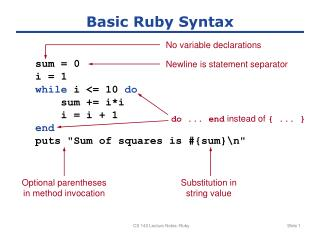Download PresentationBasic Ruby Syntax

Basic Ruby Syntax - PowerPoint PPT Presentation

Basic Ruby Syntax. No variable declarations. sum = 0 i = 1 while i <= 10 do sum += i * i i = i + 1 end puts "Sum of squares is #{sum}
". Newline is statement separator. do ... end instead of { ... }. Optional parentheses in method invocation. Substitution in string value.I am the owner, or an agent authorized to act on behalf of the owner, of the copyrighted work described.
Download PresentationBasic Ruby Syntax

Download Policy: Content on the Website is provided to you AS IS for your information and personal use and may not be sold / licensed / shared on other websites without getting consent from its author.While downloading, if for some reason you are not able to download a presentation, the publisher may have deleted the file from their server.

- - - - - - - - - - - - - - - - - - - - - - - - - - E N D - - - - - - - - - - - - - - - - - - - - - - - - - -
Presentation Transcript
1. Basic Ruby Syntax No variable declarations sum = 0 i = 1 whilei <= 10 do sum += i*i i = i + 1 end puts "Sum of squares is #{sum}\n" Newline is statement separator do ... end instead of { ... } Optional parentheses in method invocation Substitution instring value CS 142 Lecture Notes: Ruby

2. Ruby String Syntax • Single quotes (only \' and \\) 'Bill\'s "personal" book' • Double quotes (many escape sequences) "Found #{count} errors\nAborting job\n" • %q (similar to single quotes) %q<Nesting works: <b>Hello</b>> • %Q (similar to double quotes) %Q|She said "#{greeting}"\n| • “Here documents” <<END First line Second line END CS 142 Lecture Notes: Ruby

3. Arrays and Hashes x = Array.new x << 10 x = 99 y = ["Alice", 23, 7.3] x = y + y[-1] person = Hash.new person["last_name"] = "Rodriguez" person[:first_name] = "Alice" order = {"item" => "Corn Flakes", "weight" => 18} order = {:item => "Corn Flakes", :weight => 18} order = {item: "Corn Flakes", weight: 18} CS 142 Lecture Notes: Ruby

4. Variable Names and Scopes foo Local variable \$foo Global variable @foo Instance variable in object @@foo Class variable MAX_USERS “Constant” (by convention) CS 142 Lecture Notes: Ruby

5. Ruby Statements if x < 10 then ... elsifx < 20 ... else ... end while x < 10 do ... end array = [14, 22, 34, 46, 92] for value in array do ... end CS 142 Lecture Notes: Ruby

6. Factorial deffac(x) if x <= 1 then return 1 end return x*fac(x-1) end CS 142 Lecture Notes: Ruby

7. Arguments: Defaults, Variable # definc(value, amount=1) value+amount end defmax(first, *rest) max = first for x in rest do if (x > max) then max = x end end return max end CS 142 Lecture Notes: Ruby

8. Keyword Arguments defcreate_widget(size, properties) ... end create_widget(6, {:id => "table22", :class => "Cart"}) create_widget(6, :id => "table22", :class => "Cart") create_widget(6, id: "table22", class: "Cart") CS 142 Lecture Notes: Ruby

9. Blocks, Iterators, Yield odd_numbers(3) do |i| print(i, "\n") end defodd_numbers(count) number = 1 while count > 0 do yield(number) number += 2 count -= 1 end end Block: code passedto method Invoke method’s block CS 142 Lecture Notes: Ruby

10. Iterators are Reusable defsum_odd(count) sum = 0 odd_numbers(count) do |i| sum += i end return sum end defodd_numbers(count) number = 1 while count > 0 do yield(number) number += 2 count -= 1 end end CS 142 Lecture Notes: Ruby

11. Equivalent Code array = [14, 22, 34, 46, 92] for value in array do print(value, "\n") end array = [14, 22, 34, 46, 92]; array.eachdo |value| print(value, "\n") end CS 142 Lecture Notes: Ruby

12. Simple Class class Point definitialize(x, y) @x = x @y = y end defx @x end defx=(value) @x = value end end p = Point.new(3,4) puts "p.x is #{p.x}" p.x= 44 CS 142 Lecture Notes: Ruby

13. Module Example New methods available in MyClass: min, max, sort, map, select, ... classMyClass include Enumerable ... defeach ... end end CS 142 Lecture Notes: Ruby

14. CS 142 Lecture Notes: Ruby